5.4.7 Aside: Recursive Insertion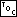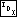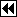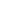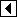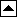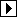In previous sections we first looked at recursive approaches because they were simpler and more elegant than iterative solutions. As it happens, the reverse is true for insertion into an AVL tree. But just for completeness, we will now design a recursive implementation of avl_probe().

Our first task in such a design is to figure out what arguments and return value the recursive core of the insertion function will have. We'll begin by considering AVL insertion in the abstract. Our existing function avl_probe() works by first moving down the tree, from the root to a leaf, then back up the tree, from leaf to root, as necessary to adjust balance factors or rebalance. In the existing iterative version, down and up movement are implemented by pushing nodes onto and popping them off from a stack. In a recursive version, moving down the tree becomes a recursive call, and moving up the tree becomes a function return.

While descending the tree, the important pieces of information are the tree itself (to allow for comparisons to be made), the current node, and the data item we're inserting. The latter two items need to be modifiable by the function, the former because the tree rooted at the node may need to be rearranged during a rebalance, and the latter because of avl_probe()'s return value.

While ascending the tree, we'll still have access to all of this information, but, to allow for adjustment of balance factors and rebalancing, we also need to know whether the subtree visited in a nested call became taller. We can use the function's return value for this.

Finally, we know to stop moving down and start moving up when we find a null pointer in the tree, which is the place for the new node to be inserted. This suggests itself naturally as the test used to stop the recursion.

Here is an outline of a recursive insertion function directly corresponding to these considerations:

```160. <Recursive insertion into AVL tree 160> =
static int probe (struct avl_table *tree, struct avl_node **p, void ***data) {
struct avl_node *y; /* The current node; shorthand for *p. */

assert (tree != NULL && p != NULL && data != NULL);

y = *p;
if (y == NULL)
{       <Found insertion point in recursive AVL insertion 161>     }
else /* y != NULL */     {       <Move down then up in recursive AVL insertion 162>     }
}
```

Parameter p is declared as a double pointer (struct avl_node **) and data as a triple pointer (void ***). In both cases, this is because C passes arguments by value, so that a function modifying one of its arguments produces no change in the value seen in the caller. As a result, to allow a function to modify a scalar, a pointer to it must be passed as an argument; to modify a pointer, a double pointer must be passed; to modify a double pointer, a triple pointer must be passed. This can result in difficult-to-understand code, so it is often advisable to copy the dereferenced argument into a local variable for read-only use, as *p is copied into y here.

When the insertion point is found, a new node is created and a pointer to it stored into *p. Because the insertion causes the subtree to increase in height (from 0 to 1), a value of 1 is then returned:

```161. <Found insertion point in recursive AVL insertion 161> =
y = *p = tree->avl_alloc->libavl_malloc (tree->avl_alloc, sizeof *y);
if (y == NULL)   {
*data = NULL;
return 0;
}

y->avl_data = **data;
*data = &y->avl_data;
y->avl_balance = 0;

tree->avl_count++;
tree->avl_generation++;

return 1;
```

This code is included in 160.

When we're not at the insertion point, we move down, then back up. Whether to move down to the left or the right depends on the value of the item to insert relative to the value in the current node y. Moving down is the domain of the recursive call to probe(). If the recursive call doesn't increase the height of a subtree of y, then there's nothing further to do, so we return immediately. Otherwise, on the way back up, it is necessary to at least adjust y's balance factor, and possibly to rebalance as well. If only adjustment of the balance factor is necessary, it is done and the return value is based on whether this subtree has changed height in the process. Rebalancing is accomplished using the same code used in iterative insertion. A rebalanced subtree has the same height as before insertion, so the value returned is 0. The details are in the code itself:

```162. <Move down then up in recursive AVL insertion 162> =
struct avl_node *w; /* New root of this subtree; replaces *p. */
int cmp;

cmp = tree->avl_compare (**data, y->avl_data, tree->avl_param);
if (cmp < 0)   {
if (probe (tree, &y->avl_link, data) == 0)
return 0;

if (y->avl_balance == +1)       {
y->avl_balance = 0;
return 0;
}
else if (y->avl_balance == 0)       {
y->avl_balance = -1;
return 1;
}     else       {         <Rebalance AVL tree after insertion in left subtree 152>       }
} else if (cmp > 0)   {
struct avl_node *r; /* Right child of y, for rebalancing. */

if (probe (tree, &y->avl_link, data) == 0)
return 0;

if (y->avl_balance == -1)       {
y->avl_balance = 0;
return 0;
}
else if (y->avl_balance == 0)       {
y->avl_balance = +1;
return 1;
}     else       {         <Rebalance AVL tree after insertion in right subtree 157>       }
} else /* cmp == 0 */   {
*data = &y->avl_data;
return 0;
}

*p = w;
return 0;
```

This code is included in 160.

Finally, we need a wrapper function to start the recursion off correctly and deal with passing back the results:

```163. <Recursive insertion into AVL tree 160> +=
/* Inserts item into tree and returns a pointer to item's address.
If a duplicate item is found in the tree,
returns a pointer to the duplicate without inserting item.
Returns NULL in case of memory allocation failure. */
void **avl_probe (struct avl_table *tree, void *item) {
void **ret = &item;

probe (tree, &tree->avl_root, &ret);

return ret;
}
```